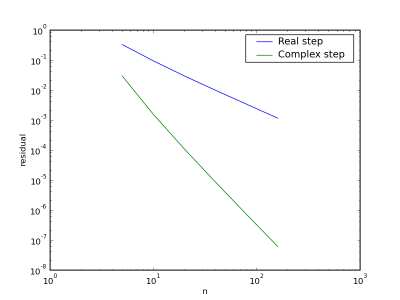## Sunday, November 23, 2008

### Is it really O(h**4)?

Over the course of a couple posts (1 and 2) we've shown that you can extend the idea of finite difference approximations to include differences in the imaginary direction. That is you can take complex steps. I presented a formula for the second derivative on a compact stencil (using only nearest neighbours) which I claimed was fourth order accurate. But is it really?

Here's a simple convergence study using cos(), whose second derivative is -cos(). We'll use python to loop over an array with the number of points per wavelength we want to use in our approximation and then calculate a residual, or error, between the analytical solution and our approximation.

for ni in n:
x = 2*pi*arange(0,ni)/(ni-1)
h = x-x
f = cos(x)
analytical_d2f = -cos(x)
# take a positive imaginary step
x_step = x + complex(0,h)
f_pc = cos(x_step)
# take a negative imaginary step
x_step = x - complex(0,h)
f_mc = cos(x_step)
# take a positive real step
x_step = x + h
f_ph = cos(x_step)
# take a negative real step
x_step = x - h
f_mh = cos(x_step)

approx_d2f_c = ( f_ph + f_mh - f_pc.real - f_mc.real)/(2*h*h)
approx_d2f_h = ( f_ph + f_mh - 2*f )/(h*h)

resid_c[i] = sqrt( sum( (analytical_d2f - approx_d2f_c)**2 ) )
resid_h[i] = sqrt( sum( (analytical_d2f - approx_d2f_h)**2 ) )
i += 1

The approximated and analytical second derivatives are shown below for 10 points per wavelength using both a standard central difference with only a real step as well as the complex step formula.The convergence behaviour for the two approximations is shown below.Obviously we're getting much better convergence with our complex step formula.